# 获取数据

# from sklearn.datasets import fetch_mldata
# from sklearn import datasets

# mnist = fetch_mldata('MNIST	original')
# mnist


from sklearn.datasets import fetch_mldata
from sklearn import datasets
import numpy as np

mnist = fetch_mldata('mnist-original', data_home = './datasets/')
mnist



{'DESCR': 'mldata.org dataset: mnist-original',
'COL_NAMES': ['label', 'data'],
'target': array([0., 0., 0., ..., 9., 9., 9.]),
'data': array([[0, 0, 0, ..., 0, 0, 0],
[0, 0, 0, ..., 0, 0, 0],
[0, 0, 0, ..., 0, 0, 0],
...,
[0, 0, 0, ..., 0, 0, 0],
[0, 0, 0, ..., 0, 0, 0],
[0, 0, 0, ..., 0, 0, 0]], dtype=uint8)}


X, y = mnist['data'], mnist['target']
print(X.shape)
print(y.shape)


(70000, 784)
(70000,)


%matplotlib inline
import matplotlib
import matplotlib.pyplot as plt

some_digit = X
some_digit_image = some_digit.reshape(28,28)

plt.imshow(some_digit_image, cmap=matplotlib.cm.binary, interpolation="nearest")
plt.axis('off')
plt.show()y


5.0


# EXTRA
def plot_digits(instances, images_per_row=10, **options):
size = 28
images_per_row = min(len(instances), images_per_row)
images = [instance.reshape(size,size) for instance in instances]
n_rows = (len(instances) - 1) // images_per_row + 1
row_images = []
n_empty = n_rows * images_per_row - len(instances)
images.append(np.zeros((size, size * n_empty)))
for row in range(n_rows):
rimages = images[row * images_per_row : (row + 1) * images_per_row]
row_images.append(np.concatenate(rimages, axis=1))
image = np.concatenate(row_images, axis=0)
plt.imshow(image, cmap = matplotlib.cm.binary, **options)
plt.axis("off")

plt.figure(figsize=(9,9))
example_images = np.r_[X[:12000:600], X[13000:30600:600], X[30600:60000:590]]
plot_digits(example_images, images_per_row=10)
# save_fig("more_digits_plot")
plt.show()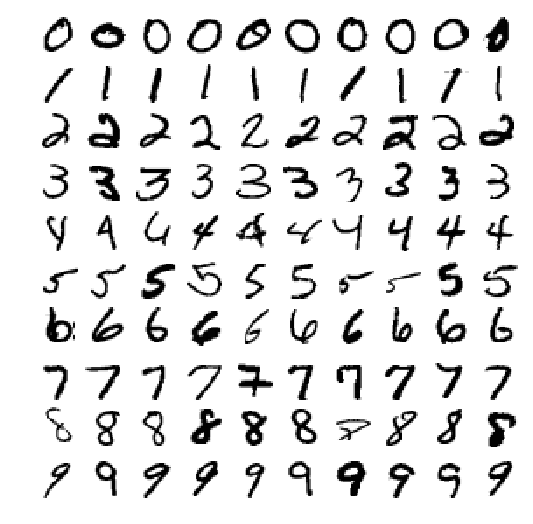X_train, X_test, y_train, y_test = X[:60000],X[60000:],y[:60000],y[60000:]


MNIST的数据是按数字大小顺序排列的，所我们先要打乱它的顺序，这样可以保证我们的交叉验证是每一次都是相似的。

import numpy as np

shuffle_index = np.random.permutation(60000)
shuffle_index


array([52603, 56601, 42625, ..., 17778, 24267, 29358])


X_train, y_train = X_train[shuffle_index],y_train[shuffle_index]


# 训练一个二分类器

# 这是一个逻辑数组，5：True, 非5：False
y_train_5 = (y_train == 5)
y_test_5 = (y_test == 5)


from sklearn.linear_model import SGDClassifier

sgd_clf = SGDClassifier(random_state = 32)
sgd_clf.fit(X_train, y_train_5)


SGDClassifier(alpha=0.0001, average=False, class_weight=None,
early_stopping=False, epsilon=0.1, eta0=0.0, fit_intercept=True,
l1_ratio=0.15, learning_rate='optimal', loss='hinge', max_iter=None,
n_iter=None, n_iter_no_change=5, n_jobs=None, penalty='l2',
power_t=0.5, random_state=32, shuffle=True, tol=None,
validation_fraction=0.1, verbose=0, warm_start=False)


sgd_clf.predict([some_digit])


array([ True])


sgd_clf = SGDClassifier(random_state = 42)
sgd_clf.fit(X_train, y_train_5)
sgd_clf.predict([some_digit])


array([ True])


# 对性能的评估

## 使用交叉验证测量准确性

from sklearn.model_selection import StratifiedKFold
from sklearn.base import clone

skfolds = StratifiedKFold(n_splits = 3, random_state = 42)
clone_clf = clone(sgd_clf)
for train_index, test_index in skfolds.split(X_train, y_train_5):
X_train_folds = X_train[train_index]
y_train_folds = (y_train_5[train_index])
X_test_fold = X_train[test_index]
y_test_fold = (y_train_5[test_index])
clone_clf.fit(X_train_folds, y_train_folds)
y_pred = clone_clf.predict(X_test_fold)
n_correct = sum(y_pred == y_test_fold)
print(n_correct / len(y_pred))


0.9612

0.9531

0.9688


from sklearn.model_selection import cross_val_score

cross_val_score(sgd_clf, X_train, y_train_5, cv = 3, scoring = "accuracy")



array([0.9612, 0.9531, 0.9688])


from sklearn.base import BaseEstimator
# 这个模型的预测的策略就是将所有的数据都认为是'非5'
class Never5Classifier(BaseEstimator):
def fit(self,X,y=None):
pass
def predict(self,X):
return np.zeros((len(X),1), dtype=bool)


never_5_clf = Never5Classifier()
cross_val_score(never_5_clf, X_train, y_train_5, cv = 3, scoring = "accuracy")


array([0.90815, 0.9124 , 0.9084 ])


## 混淆矩阵

from sklearn.model_selection import cross_val_predict

y_train_pred = cross_val_predict(sgd_clf, X_train, y_train_5, cv = 3)

from sklearn.metrics import confusion_matrix

confusion_matrix(y_train_5, y_train_pred)


array([[54306,   273],
[ 2065,  3356]], dtype=int64)


# confusion_matrix(y_train_5, y_train_perfect_predictions)


$precision = \frac{TP}{TP + FP} \tag{3-1}$

$recall = \frac{TP}{TP+FN} \tag{3-2}$

$FN$是假反例的数目。

from sklearn.metrics import precision_score, recall_score

print(precision_score(y_train_5, y_train_pred))
print(recall_score(y_train_5, y_train_pred))


0.924772664645908
0.6190739715919572


$F1 = \frac{2}{\frac{1}{precision}+\frac{1}{recall}} = 2 \times \frac{precision \times recall}{precision + recall} = \frac{TP}{TP + \frac{FN+FP}{2}} \tag{3-3}$

from sklearn.metrics import f1_score

f1_score(y_train_5, y_train_pred)

0.7416574585635358


F1支持那些有着相近准确率和召回率的分类（意思是只有当准确率和召回率一样大的时个，F1值才会大）。但并不是所的时候，我们都关心F1值，有时候我们只关心准确率（precision），或者有时候我们只关心召回率（recall）。

### 准备率/召回率之间的折中

y_scores = sgd_clf.decision_function([some_digit])
y_scores


array([15905.22111141])

threshold = 0
y_some_digit_pred = (y_scores > threshold)
y_some_digit_pred


array([ True])

y_scores = cross_val_predict(sgd_clf, X_train,y_train_5,cv=3,
method = "decision_function")

from sklearn.metrics import precision_recall_curve
precisions, recalls, thresholds = precision_recall_curve(y_train_5, y_scores)

def plot_precision_recall_vs_threshold(precisions, recalls, thresholds):
plt.plot(thresholds, precisions[:-1], "b--", label = "Precision")
plt.plot(thresholds, recalls[:-1], "g-", label = "Recall")
plt.xlabel("Threshold")
plt.legend(loc="upper left")
plt.ylim([0,1.1])

plot_precision_recall_vs_threshold(precisions,recalls,thresholds)
plt.grid()
plt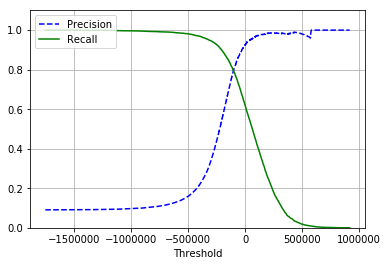### ROC曲线

from sklearn.metrics import roc_curve
fpr, tpr, thresholds = roc_curve(y_train_5, y_scores)

def plot_roc_curve(fpr, tpr, label = None):
plt.plot(fpr,tpr, linewidth = 2, label = label)
plt.plot([0,1],[0,1],'k--')
plt.axis([0,1,0,1])
plt.xlabel('False Positive Rate')
plt.ylabel('True Positive Rate')

plot_roc_curve(fpr,tpr)
plt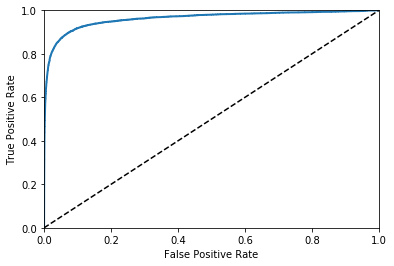from sklearn.metrics import roc_auc_score
roc_auc_score(y_train_5,y_scores)

0.9623990527630832

from sklearn.ensemble import RandomForestClassifier

forest_clf = RandomForestClassifier(random_state = 42)
y_probas_forest = cross_val_predict(forest_clf, X_train, y_train_5, cv=3, method = "predict_proba")

y_scores_forest = y_probas_forest[:,1]
fpr_forest,	tpr_forest,	thresholds_forest=roc_curve(y_train_5,y_scores_forest)
plt.plot(fpr,tpr,"b:",label="SGD")
plot_roc_curve(fpr_forest,tpr_forest,"Random Forest")
plt.legend(loc="bottom	right")
plt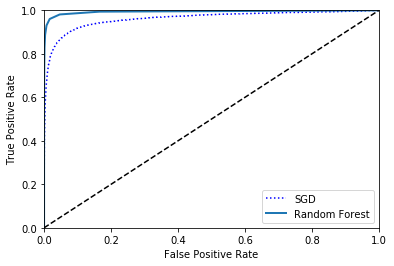# 将概率大于0.5的，置为true, 否则为false
print(precision_score(y_train_5, y_scores_forest > 0.5))
print(recall_score(y_train_5, y_scores_forest > 0.5))


0.9844298245614035
0.8280760007378712


# 多类分类

Scikit-Learn可以探测出你想使用一个二分类器去完成多分类的任务，它会自动地执行OvA（除了SVM分类器，它使用OvO）。让我们试一下SGDClassifier

sgd_clf.fit(X_train, y_train)
sgd_clf.predict([some_digit])

array([5.])


some_digit_scores = sgd_clf.decision_function([some_digit])
some_digit_scores

array([[-253639.46707377, -425198.63904333, -354213.80127786,
-229676.13263264, -376404.48500382,   15905.22111141,
-564592.12430579, -194289.65607053, -748913.30208666,
-597652.52038338]])


np.argmax(some_digit_scores)

5

sgd_clf.classes_

array([0., 1., 2., 3., 4., 5., 6., 7., 8., 9.])


from sklearn.multiclass import OneVsOneClassifier

ovo_clf = OneVsOneClassifier(SGDClassifier(random_state=42))
ovo_clf.fit(X_train, y_train)
ovo_clf.predict([some_digit])

array([5.])


forest_clf.fit(X_train,y_train)
forest_clf.predict([some_digit])

array([5.])


forest_clf.predict_proba([some_digit])

array([[0. , 0. , 0. , 0. , 0. , 0.9, 0. , 0. , 0.1, 0. ]])


cross_val_score(sgd_clf, X_train, y_train,cv = 3, scoring = "accuracy")

array([0.86002799, 0.8760438 , 0.88093214])


from sklearn.preprocessing import StandardScaler

scaler = StandardScaler()
X_train_scaled = scaler.fit_transform(X_train.astype(np.float64))
cross_val_score(sgd_clf, X_train_scaled, y_train, cv = 3, scoring="accuracy")

array([0.9080184 , 0.91049552, 0.91043657])


# 误差分析

y_train_pred = cross_val_predict(sgd_clf, X_train_scaled, y_train, cv = 3)
conf_mx = confusion_matrix(y_train, y_train_pred)
conf_mx

array([[5739,    3,   22,    8,    9,   50,   43,    7,   38,    4],
[   2, 6451,   50,   23,    6,   46,    5,   14,  133,   12],
[  58,   38, 5348,   87,   76,   26,   83,   56,  169,   17],
[  50,   40,  134, 5300,    2,  267,   37,   64,  140,   97],
[  25,   26,   36,    7, 5356,    9,   54,   32,   83,  214],
[  68,   37,   34,  179,   74, 4617,  106,   30,  171,  105],
[  35,   21,   42,    2,   39,   98, 5630,    6,   44,    1],
[  27,   18,   66,   27,   52,   10,    7, 5793,   17,  248],
[  58,  150,   68,  140,   16,  156,   51,   29, 5050,  133],
[  43,   29,   24,   84,  158,   36,    3,  194,   83, 5295]],
dtype=int64)


plt.matshow(conf_mx, cmap = plt.cm.gray)
plt.show()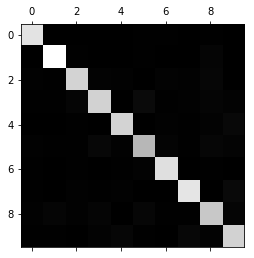row_sums = conf_mx.sum(axis=1, keepdims=True)
norm_conf_mx = conf_mx / row_sums

np.fill_diagonal(norm_conf_mx, 0)
plt.matshow(norm_conf_mx, cmap = plt.cm.gray)
plt.show()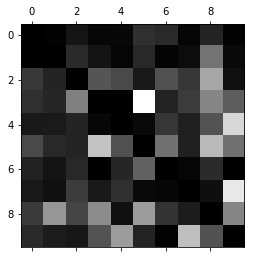cl_a, cl_b = 3, 5
X_aa = X_train[(y_train == cl_a) & (y_train_pred == cl_a)]
X_ab = X_train[(y_train == cl_a) & (y_train_pred == cl_b)]
X_ba = X_train[(y_train == cl_b) & (y_train_pred == cl_a)]
X_bb = X_train[(y_train == cl_b) & (y_train_pred == cl_b)]

plt.figure(figsize=(8,8))
plt.subplot(221); plot_digits(X_aa[:25], images_per_row=5)
plt.subplot(222); plot_digits(X_ab[:25], images_per_row=5)
plt.subplot(223); plot_digits(X_ba[:25], images_per_row=5)
plt.subplot(224); plot_digits(X_bb[:25], images_per_row=5)
# save_fig("error_analysis_digits_plot")
plt.show()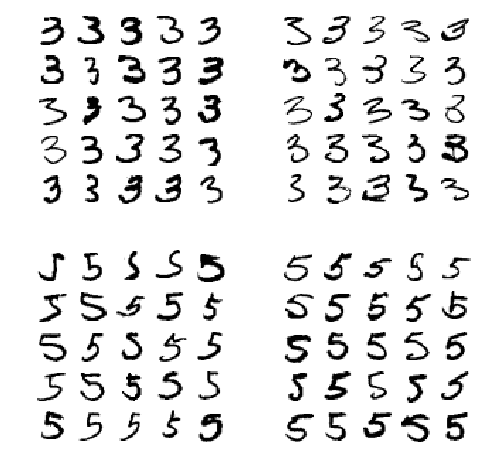# 多标签分类

from sklearn.neighbors import KNeighborsClassifier
y_train_large = (y_train >=7)
y_train_odd = (y_train % 2 == 1)
y_multilabel = np.c_[y_train_large, y_train_odd]

knn_clf = KNeighborsClassifier()
knn_clf.fit(X_train, y_multilabel)

KNeighborsClassifier(algorithm='auto', leaf_size=30, metric='minkowski',
metric_params=None, n_jobs=None, n_neighbors=5, p=2,
weights='uniform')


knn_clf.predict([some_digit])

array([[False,  True]])


# y_train_knn_pred = cross_val_predict(knn_clf, X_train, y_train, cv = 3)
# f1_score(y_train, y_train_knn_pred, average="macro")


# 多输出分类

noise = np.random.randint(0, 100, (len(X_train), 784))
X_train_mod = X_train + noise
noise = np.random.randint(0, 100, (len(X_test), 784))
X_test_mod = X_test + noise
y_train_mod = X_train
y_test_mod = X_test

def plot_digit(data):
image = data.reshape(28, 28)
plt.imshow(image, cmap = matplotlib.cm.binary,
interpolation="nearest")
plt.axis("off")

some_index = 5500

plt.subplot(121); plot_digit(X_test_mod[some_index])
plt.subplot(122); plot_digit(y_test_mod[some_index])
# save_fig("noisy_digit_example_plot")
plt.show()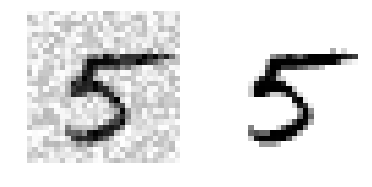knn_clf.fit(X_train_mod, y_train_mod)
clean_digit = knn_clf.predict([X_test_mod[some_index]])
plot_digit(clean_digit)
# save_fig("cleaned_digit_example_plot")posted @ 2018-11-03 19:52  EndlessCoding  阅读(52628)  评论(6编辑  收藏  举报